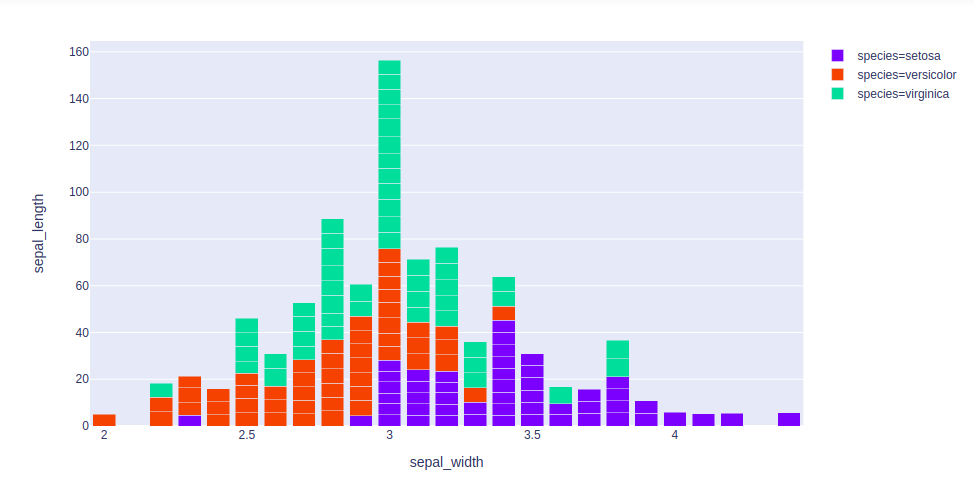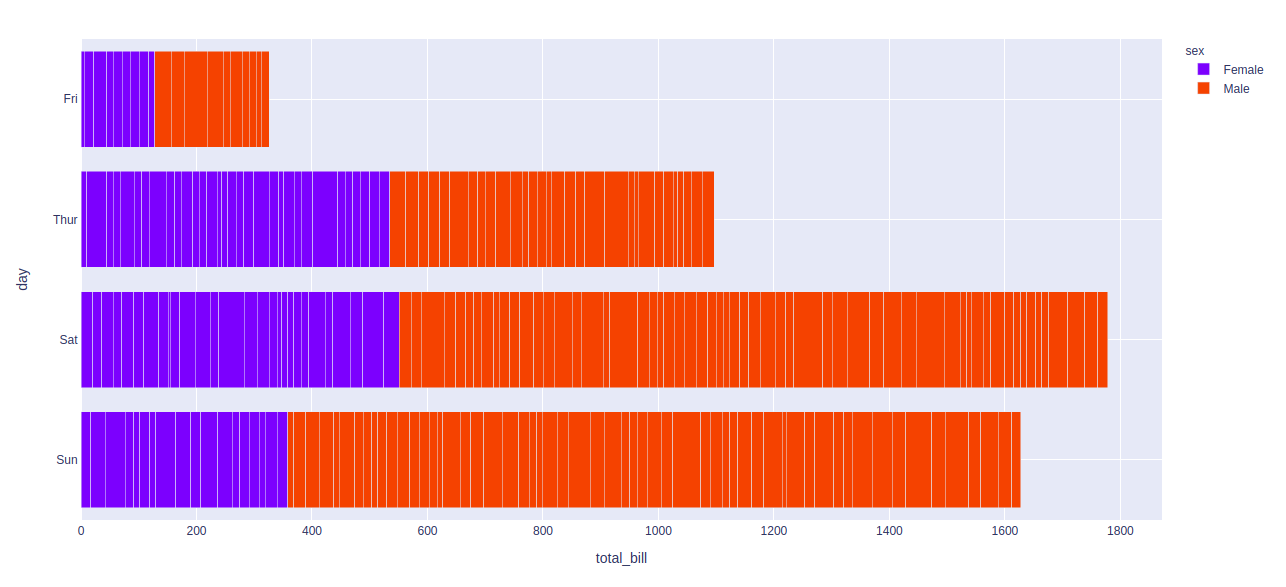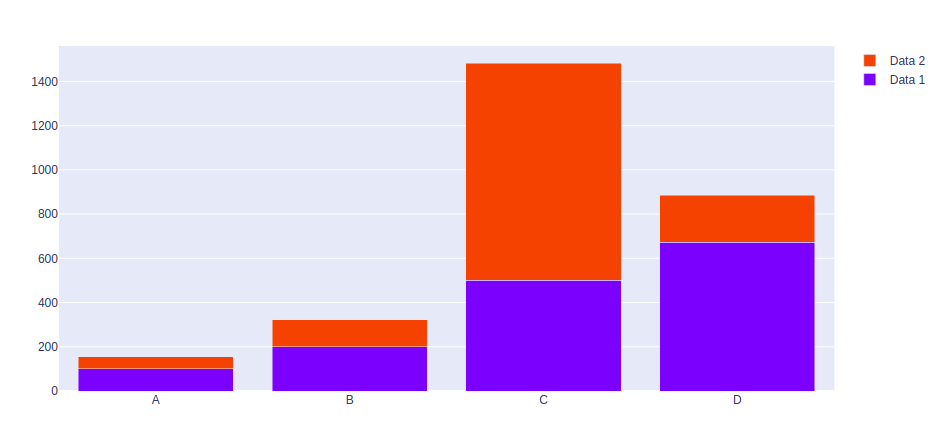Open in App
Not now

# How to create Stacked bar chart in Python-Plotly?

• Last Updated : 29 Oct, 2020

Plotly is a Python library which is used to design graphs, especially interactive graphs. It can plot various graphs and charts like histogram, barplot, boxplot, spreadplot and many more. It is mainly used in data analysis as well as financial analysis. plotly is an interactive visualization library.

## Stack bar chart

A stacked bar chart or  graph is a chart that uses bars to demonstrate comparisons between categories of data, but with ability to impart and compare parts of a whole. Each bar in the chart represents a whole and segments which represent different parts or categories of that whole.

Example 1: Using iris dataset

## Python3

 `import` `plotly.express as px` `df ``=` `px.data.iris()` `fig ``=` `px.bar(df, x``=``"sepal_width"``, y``=``"sepal_length"``, color``=``"species"``,``            ``hover_data``=``[``'petal_width'``], barmode ``=` `'stack'``)` `fig.show()`

Output:Example 2: Using tips dataset

## Python3

 `import` `plotly.express as px` `df ``=` `px.data.tips()` `fig ``=` `px.bar(df, x``=``"total_bill"``, y``=``"day"``,``             ``color``=``"sex"``, barmode ``=` `'stack'``)` `fig.show()`

Output:Example 3: Using graph_objects class

## Python3

 `import` `plotly.graph_objects as px``import` `numpy as np`  `# creating random data through randomint``# function of numpy.random``np.random.seed(``42``)` `random_x``=` `np.random.randint(``1``,``101``,``100``)``random_y``=` `np.random.randint(``1``,``101``,``100``)` `x ``=` `[``'A'``, ``'B'``, ``'C'``, ``'D'``]` `plot ``=` `px.Figure(data``=``[go.Bar(``    ``name ``=` `'Data 1'``,``    ``x ``=` `x,``    ``y ``=` `[``100``, ``200``, ``500``, ``673``]``   ``),``                       ``go.Bar(``    ``name ``=` `'Data 2'``,``    ``x ``=` `x,``    ``y ``=` `[``56``, ``123``, ``982``, ``213``]``   ``)``])` `plot.update_layout(barmode``=``'stack'``)``                 ` `plot.show()`

Output:My Personal Notes arrow_drop_up